# 9 6 Surface Area of Prisms and Cylinders

• Slides: 42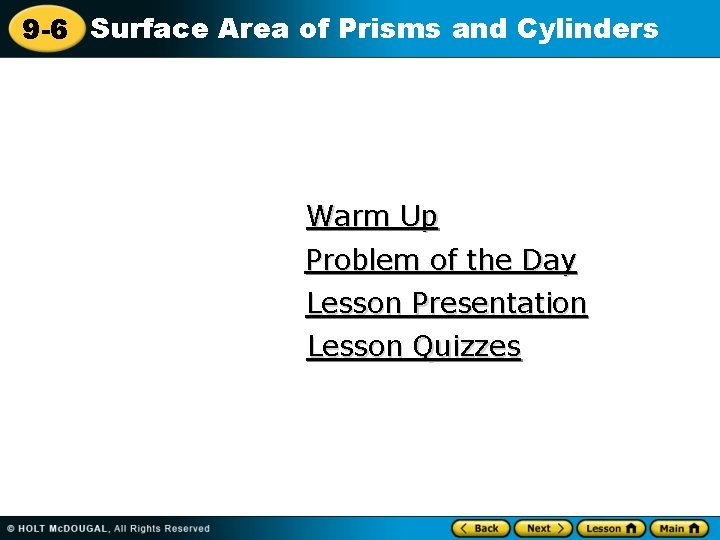9 -6 Surface Area of Prisms and Cylinders Warm Up Problem of the Day Lesson Presentation Lesson Quizzes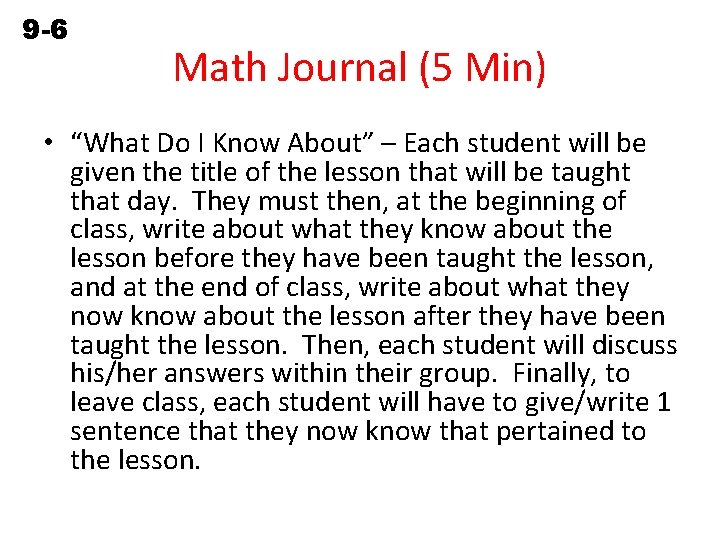9 -6 Surface Area of Prisms and Cylinders Math Journal (5 Min) • “What Do I Know About” – Each student will be given the title of the lesson that will be taught that day. They must then, at the beginning of class, write about what they know about the lesson before they have been taught the lesson, and at the end of class, write about what they now know about the lesson after they have been taught the lesson. Then, each student will discuss his/her answers within their group. Finally, to leave class, each student will have to give/write 1 sentence that they now know that pertained to the lesson.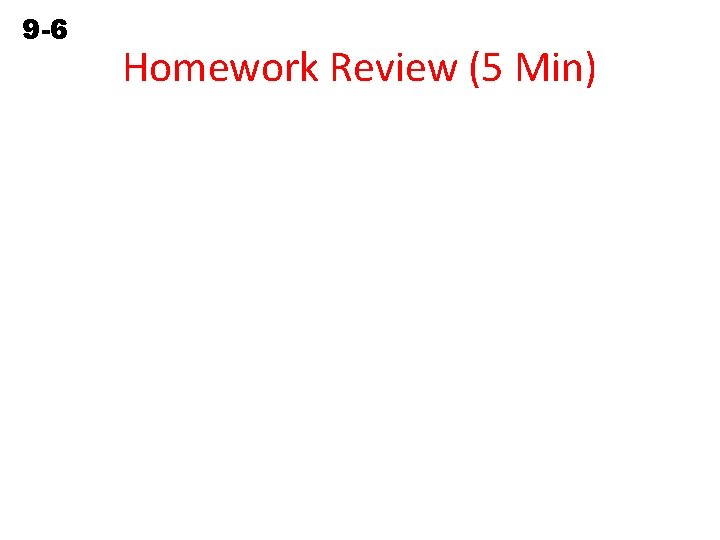9 -6 Surface Area of Prisms and Cylinders Homework Review (5 Min)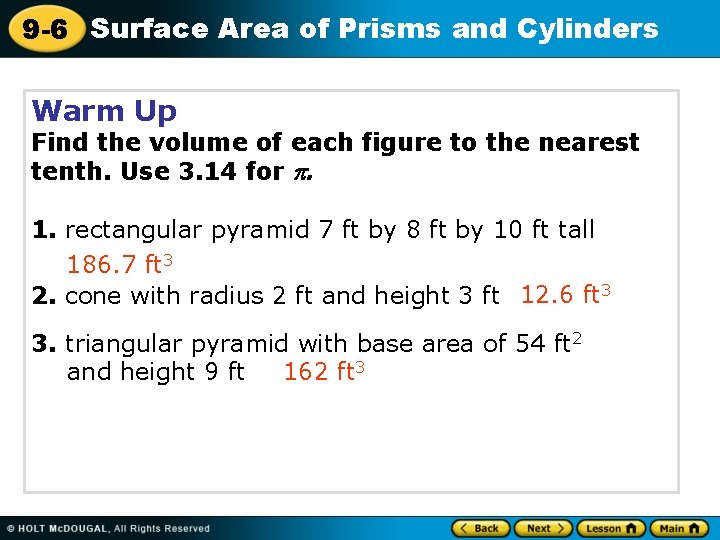9 -6 Surface Area of Prisms and Cylinders Warm Up Find the volume of each figure to the nearest tenth. Use 3. 14 for . 1. rectangular pyramid 7 ft by 8 ft by 10 ft tall 186. 7 ft 3 2. cone with radius 2 ft and height 3 ft 12. 6 ft 3 3. triangular pyramid with base area of 54 ft 2 162 ft 3 and height 9 ft9 -6 Surface Area of Prisms and Cylinders Problem of the Day The volume of a 10 -meter-tall square pyramid is 120 m 3. What is the length of each side of the base? 6 m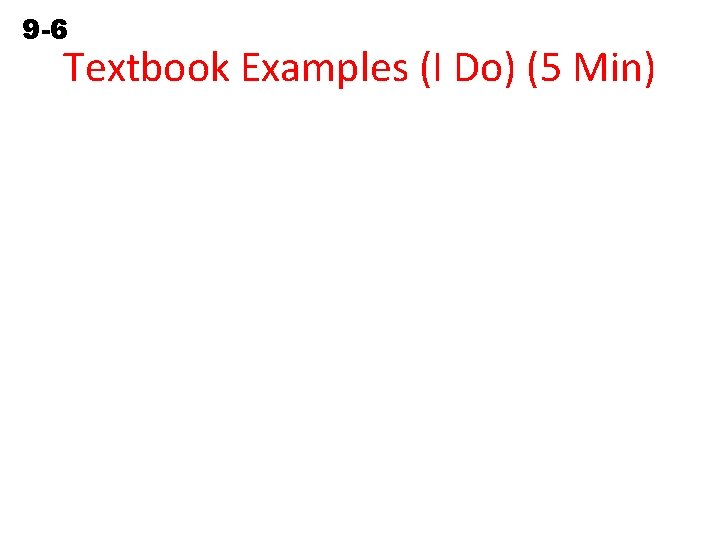9 -6 Surface Area of Prisms and Cylinders Textbook Examples (I Do) (5 Min)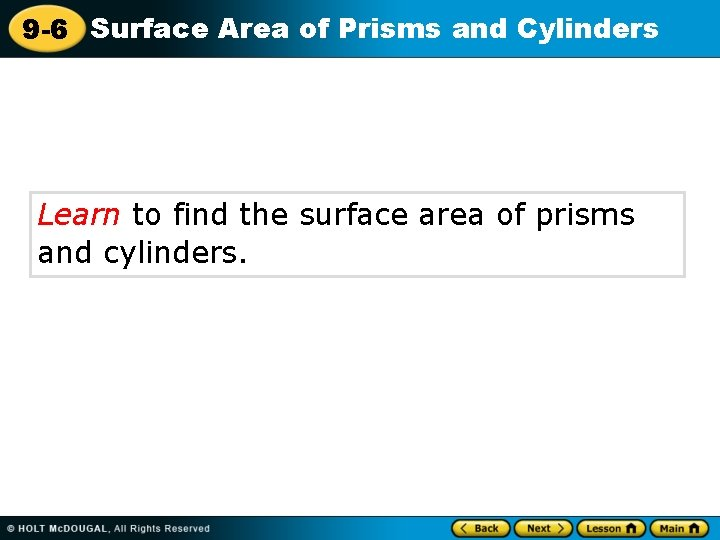9 -6 Surface Area of Prisms and Cylinders Learn to find the surface area of prisms and cylinders.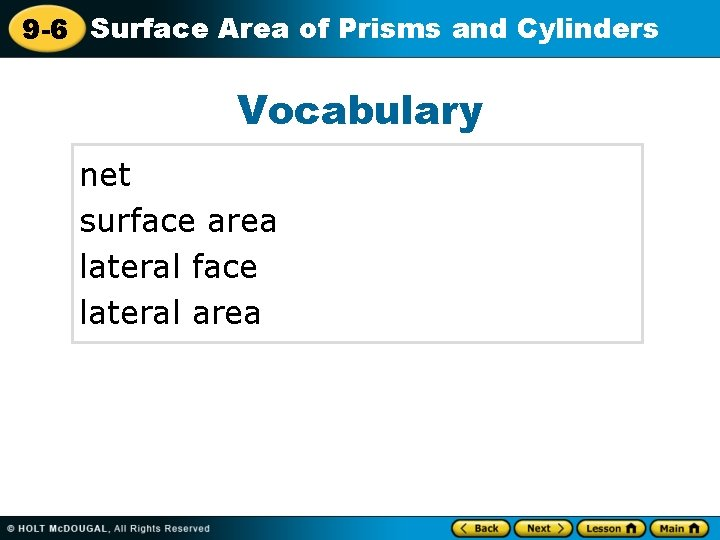9 -6 Surface Area of Prisms and Cylinders Vocabulary net surface area lateral face lateral area9 -6 Surface Area of Prisms and Cylinders If you remove the surface from a threedimensional figure and lay it out flat, the pattern you make is called a net. Nets allow you to see all the surfaces of a solid at one time. You can use nets to help you find the surface area of a three-dimensional figure. Surface area is the sum of the areas of all of the surfaces of a figure expressed in square units.9 -6 Surface Area of Prisms and Cylinders The lateral faces of a prism are parallelograms that connect the bases. The lateral area of a prism is the sum of the areas of the lateral faces.9 -6 Surface Area of Prisms and Cylinders9 -6 Surface Area of Prisms and Cylinders Additional Example 1: Finding the Surface Area of a Prism Find the surface area of the prism. S = 2 B + Ph Use the formula. S = 2(7)(15) + (44)(9) Substitute. P = 2(7) + 2(15) = 44 S = 210 + 396 S = 606 The surface area of the prism is 606 in 2.9 -6 Surface Area of Prisms and Cylinders Check It Out: Example 1 Find the surface area of the prism. S = 2 B + Ph Use the formula. 10 in. 8 in. S = 2(8)(14) + (44)(10) Substitute. P = 2(8) + 2(14) = 44 S = 224 + 440 S = 664 The surface area of the prism is 664 in 2. 14 in.9 -6 Surface Area of Prisms and Cylinders The lateral area of a cylinder is the curved surface that connects the two bases. The net of a cylinder can be drawn so that the lateral area forms a rectangle with the same height as the cylinder. The length of the rectangle is equal to the circumference of the base of the height.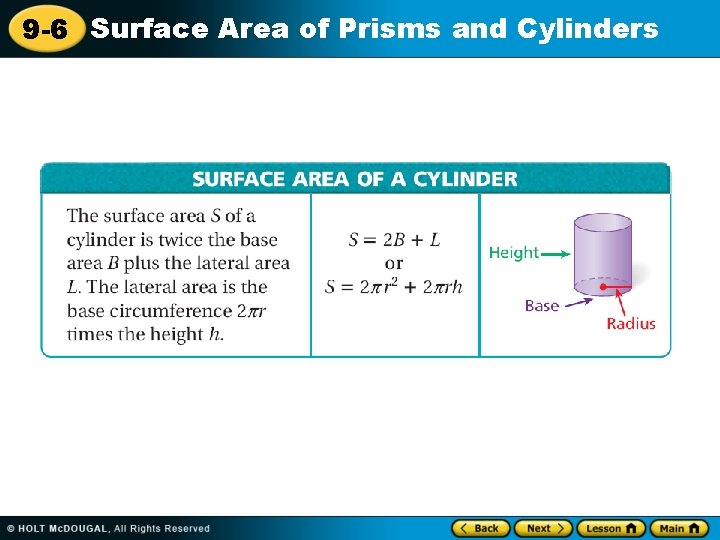9 -6 Surface Area of Prisms and Cylinders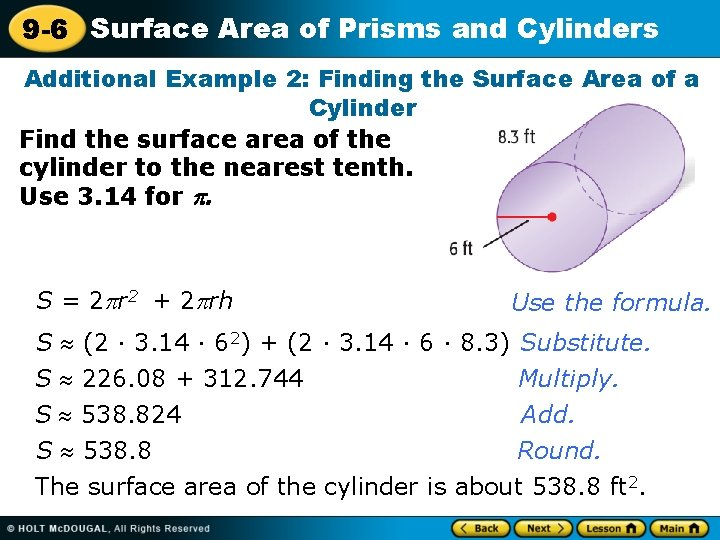9 -6 Surface Area of Prisms and Cylinders Additional Example 2: Finding the Surface Area of a Cylinder Find the surface area of the cylinder to the nearest tenth. Use 3. 14 for . S = 2 r 2 + 2 rh Use the formula. S (2 · 3. 14 · 62) + (2 · 3. 14 · 6 · 8. 3) Substitute. S 226. 08 + 312. 744 Multiply. Add. S 538. 824 Round. S 538. 8 The surface area of the cylinder is about 538. 8 ft 2.9 -6 Surface Area of Prisms and Cylinders Check It Out: Example 2 Find the surface area of the cylinder to the nearest tenth. Use 3. 14 for . 20 ft 9 ft S = 2 r 2 + 2 rh Use the formula. S (2 · 3. 14 · 92) + (2 · 3. 14 · 9 · 20) Substitute. S 508. 68 + 1130. 4 Multiply. Add. S 1, 639. 08 Round. S 1, 639. 1 The surface area of the cylinder is about 1, 639. 1 ft 2.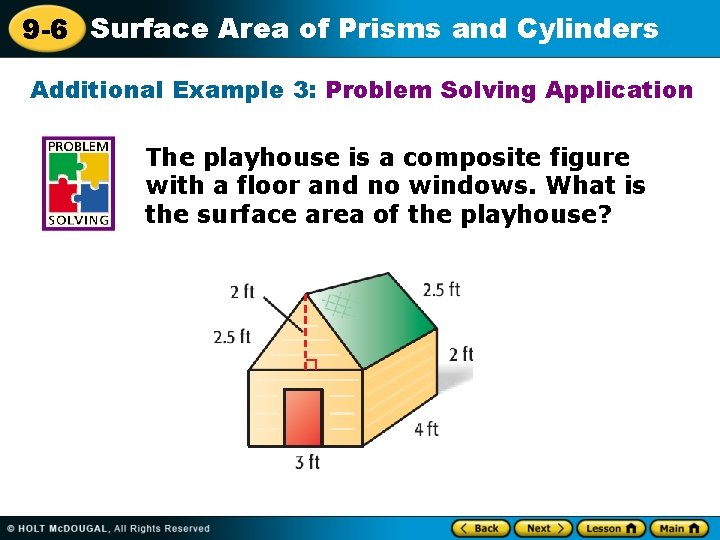9 -6 Surface Area of Prisms and Cylinders Additional Example 3: Problem Solving Application The playhouse is a composite figure with a floor and no windows. What is the surface area of the playhouse?9 -6 Surface Area of Prisms and Cylinders Additional Example 3 Continued 1 Understand the Problem • The playhouse is a rectangular prism and triangular prism. • The base of the playhouse is 3 ft by 4 ft and the height is 2 ft. • The base of the roof is 3 by 2 ft. The height of the prism is 4 ft.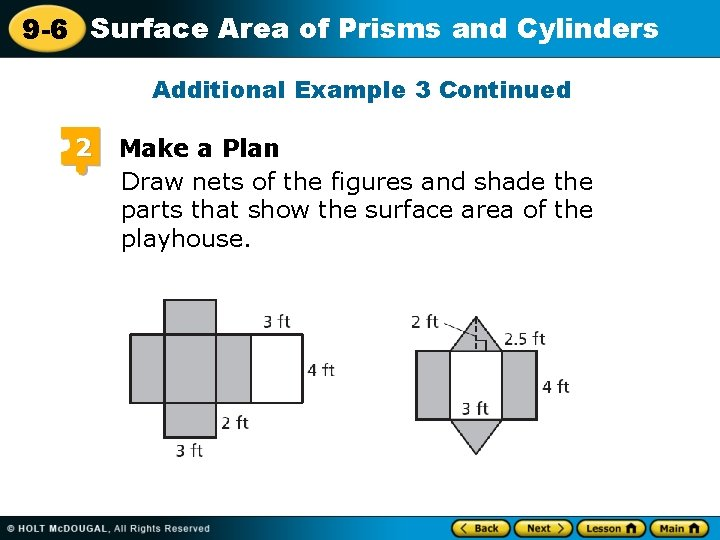9 -6 Surface Area of Prisms and Cylinders Additional Example 3 Continued 2 Make a Plan Draw nets of the figures and shade the parts that show the surface area of the playhouse.9 -6 Surface Area of Prisms and Cylinders 3 Additional Example 3 Continued Solve Find the surface are of the rectangular prism. S = B + Ph Use only one base. = (3)(4) + (14)(2) = 40 ft 2 Find the surface area of the triangular prism. S = 2 B + Ph – lw = 2(1 bh) + Ph – lw Subtract the area of the bottom of the triangular prism. 2 = 2(1 )(3)(2) + (8)(4) – (3)(4) 2 = 6 + 32 – 12 = 269 -6 Surface Area of Prisms and Cylinders Additional Example 3 Continued Add to find the total surface area: 40 + 26 = 66. The surface area of the playhouse is 66 ft 2. 4 Look Back The surface area of the playhouse should be less than the surface area of a rectangular prism with the same base and height of 4 ft. S = 2 B + Ph = 2(3)(4) + (14)(4) = 80 66 ft 2 is less than 80 ft 2 so the answer is reasonable.9 -6 Surface Area of Prisms and Cylinders Check It Out: Example 3 The playhouse is a composite figure with a floor and no windows. What is the surface area of the playhouse? 5 ft 4 ft 8 ft 6 ft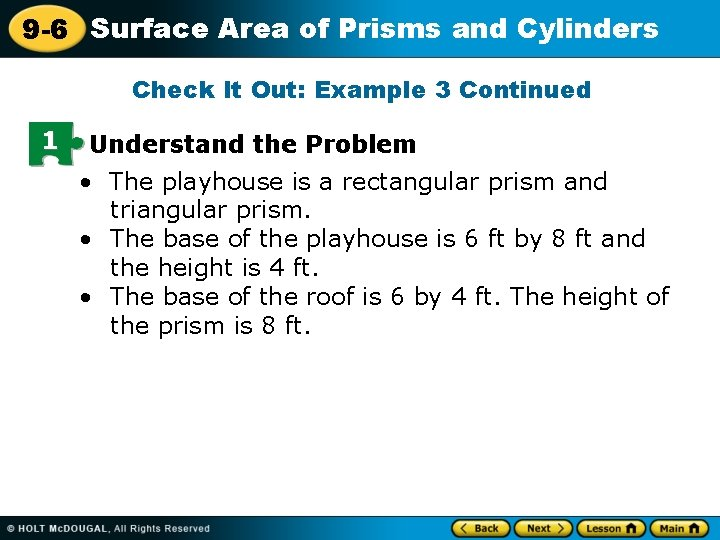9 -6 Surface Area of Prisms and Cylinders Check It Out: Example 3 Continued 1 Understand the Problem • The playhouse is a rectangular prism and triangular prism. • The base of the playhouse is 6 ft by 8 ft and the height is 4 ft. • The base of the roof is 6 by 4 ft. The height of the prism is 8 ft.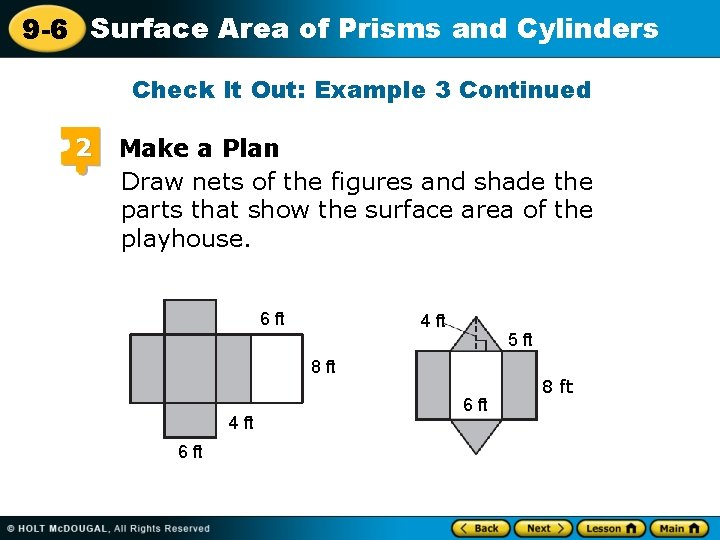9 -6 Surface Area of Prisms and Cylinders Check It Out: Example 3 Continued 2 Make a Plan Draw nets of the figures and shade the parts that show the surface area of the playhouse. 6 ft 4 ft 5 ft 8 ft 4 ft 6 ft 88 ftft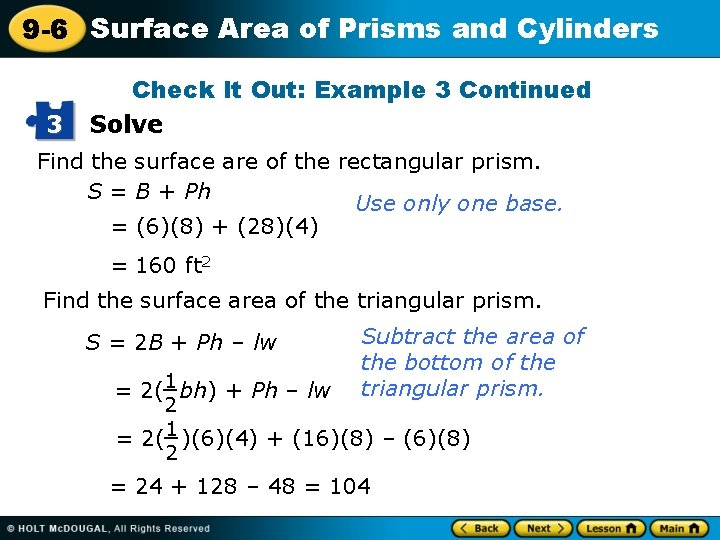9 -6 Surface Area of Prisms and Cylinders 3 Check It Out: Example 3 Continued Solve Find the surface are of the rectangular prism. S = B + Ph Use only one base. = (6)(8) + (28)(4) = 160 ft 2 Find the surface area of the triangular prism. S = 2 B + Ph – lw = 2(1 bh) + Ph – lw Subtract the area of the bottom of the triangular prism. 2 = 2(1 )(6)(4) + (16)(8) – (6)(8) 2 = 24 + 128 – 48 = 104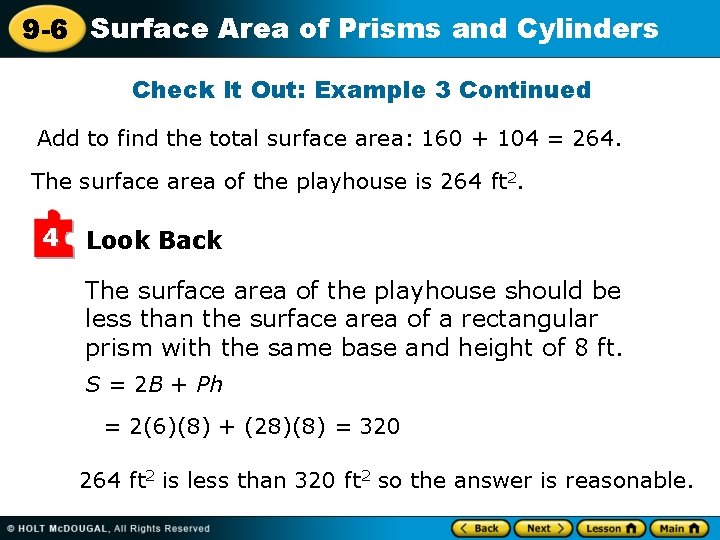9 -6 Surface Area of Prisms and Cylinders Check It Out: Example 3 Continued Add to find the total surface area: 160 + 104 = 264. The surface area of the playhouse is 264 ft 2. 4 Look Back The surface area of the playhouse should be less than the surface area of a rectangular prism with the same base and height of 8 ft. S = 2 B + Ph = 2(6)(8) + (28)(8) = 320 264 ft 2 is less than 320 ft 2 so the answer is reasonable.9 -6 Surface Area of Prisms and Cylinders Class work Problems (We Do) (10 Min) • Pg. 390 -391 (1 -5)9 -6 Surface Area of Prisms and Cylinders9 -6 Surface Area of Prisms and Cylinders9 -6 Surface Area of Prisms and Cylinders Small Group CW(Yall Do) (10 Min) • Pg. 390 -391 (6 -14 EOE)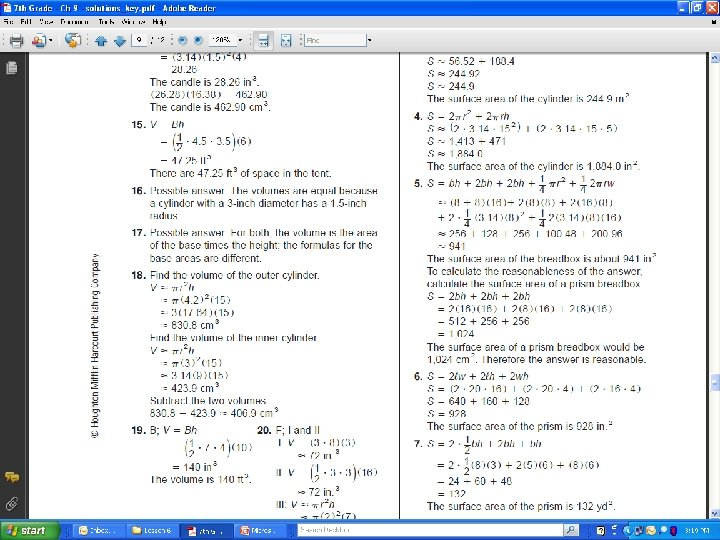9 -6 Surface Area of Prisms and Cylinders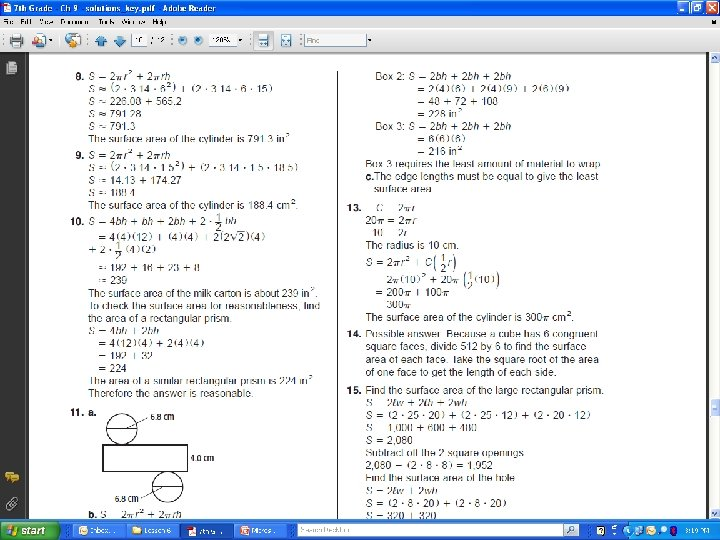9 -6 Surface Area of Prisms and Cylinders9 -6 Surface Area of Prisms and Cylinders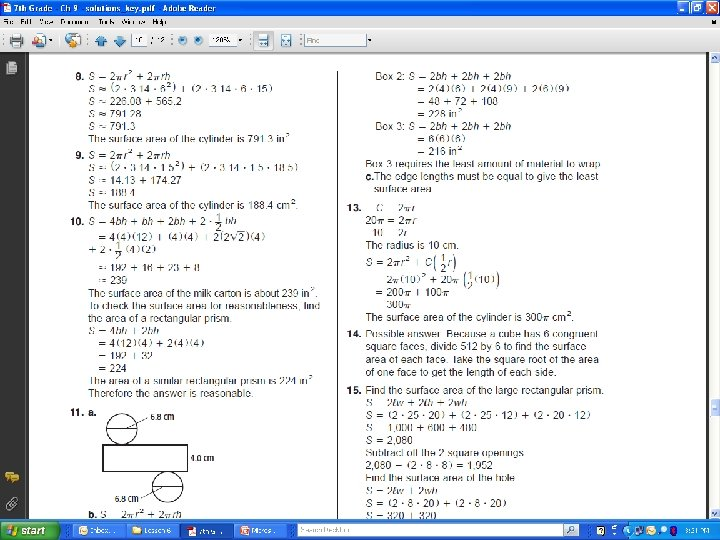9 -6 Surface Area of Prisms and Cylinders9 -6 Surface Area of Prisms and Cylinders9 -6 Surface Area of Prisms and Cylinders Homework (You Do) (10 Min) • Pg. 390 -391 (7, 9, 11, 13, 15 odd)9 -6 Surface Area of Prisms and Cylinders Math Journal (5 Min) • “What Do I Know About” – Each student will be given the title of the lesson that will be taught that day. They must then, at the beginning of class, write about what they know about the lesson before they have been taught the lesson, and at the end of class, write about what they now know about the lesson after they have been taught the lesson. Then, each student will discuss his/her answers within their group. Finally, to leave class, each student will have to give/write 1 sentence that they now know that pertained to the lesson.9 -6 Surface Area of Prisms and Cylinders Lesson Quizzes Standard Lesson Quiz for Student Response Systems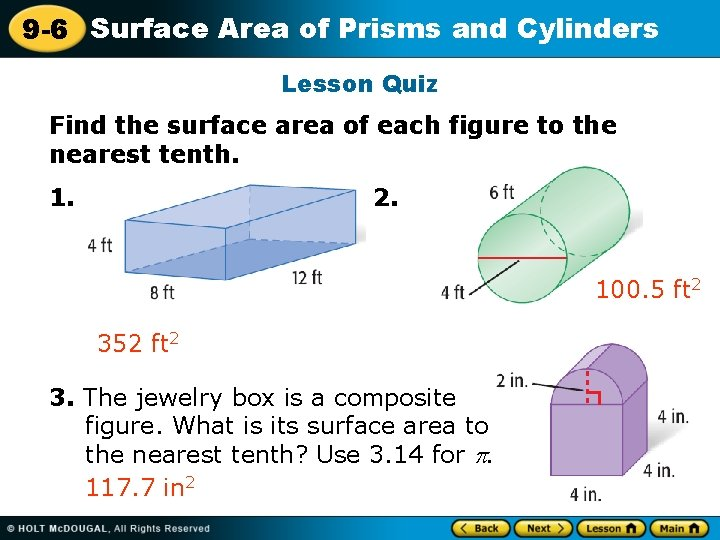9 -6 Surface Area of Prisms and Cylinders Lesson Quiz Find the surface area of each figure to the nearest tenth. 1. 2. 100. 5 ft 2 352 ft 2 3. The jewelry box is a composite figure. What is its surface area to the nearest tenth? Use 3. 14 for 117. 7 in 2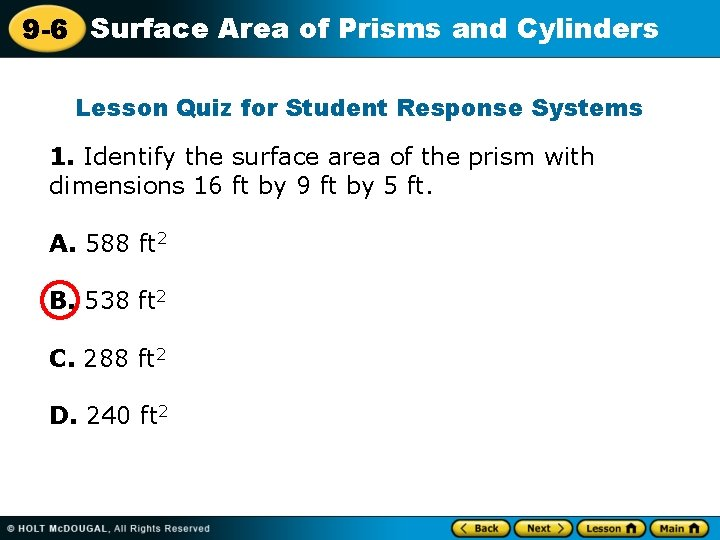9 -6 Surface Area of Prisms and Cylinders Lesson Quiz for Student Response Systems 1. Identify the surface area of the prism with dimensions 16 ft by 9 ft by 5 ft. A. 588 ft 2 B. 538 ft 2 C. 288 ft 2 D. 240 ft 2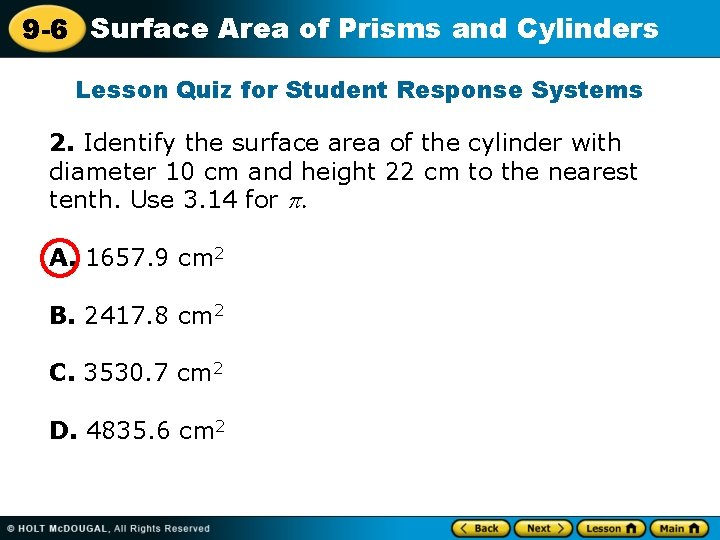9 -6 Surface Area of Prisms and Cylinders Lesson Quiz for Student Response Systems 2. Identify the surface area of the cylinder with diameter 10 cm and height 22 cm to the nearest tenth. Use 3. 14 for . A. 1657. 9 cm 2 B. 2417. 8 cm 2 C. 3530. 7 cm 2 D. 4835. 6 cm 2